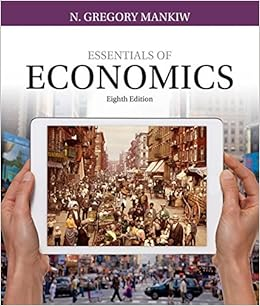# Homework E on Intermediate Macroeconomic Theory - Fiscal...

• Homework Help
• Ramala
• 2

This preview shows page 1 - 2 out of 2 pages.

##### We have textbook solutions for you!
The document you are viewing contains questions related to this textbook.The document you are viewing contains questions related to this textbook.
Chapter 24 / Exercise 1
Essentials of Economics
MankiwExpert Verified
Fiscal and Monetary Policy in the Growth Model Additional Homework Problems ECON 3133 Dr. Keen Problems 1. Consider a closed economy in which net exports, X – IM, equals 0. Suppose that consumption is insensitive to the interest rate, but the share of investment in GDP rises by 2 percent for every 1 percent decline in the interest rate. a. By how much does investment rise as a share of GDP if government purchases decrease by 4 percent of GDP? b. By how much does the interest rate change? 2. Suppose that output is equal to its potential at 4,000 and the equilibrium interest rate is 0.05. Money demand is given by M D = (0.3×Y – 4,000×R)×P. Money supply, M S , is set at 1,000 by the Federal Reserve. a. What price level is required for equilibrium in the money market? b. Suppose the Federal Reserve increases the money supply by 100. What is the new price level? What is the percentage change in the money supply and the price level? c. Starting with a money supply of 1,000 and a price level of 1.0, how does an increase in the interest rate from 0.05 to 0.10 affect the equilibrium price level? What could cause
##### We have textbook solutions for you!
The document you are viewing contains questions related to this textbook.The document you are viewing contains questions related to this textbook.
Chapter 24 / Exercise 1
Essentials of Economics
MankiwExpert Verified
•••